﻿ Function Identification — Keep Talking and Nobody Explodes Module## On the Subject of Function Identification

Does this module function as intended?

This needy module will show a random graph of a function upon activation. The defuser’s goal is to find the type of the function from the graph and submit it.

### Controlling the module

• Use the left and right arrows at the bottom of the module to scroll through different types of functions.

The module will deactivate if you guess the function type correctly. Upon an incorrect submit, you will get a strike. The module will not deactivate.

### Important information

• Only the types of functions listed below can appear on the module.
• Logarithmic functions are always in the form of y = logax, where a > 1, to avoid confusion between logarithmic graphs and exponential graphs.
• Exponential functions are never in the form of y = -ax, where 0 ≤ a ≤ 1, to avoid confusion between logarithmic graphs and exponential graphs.

### Types of functions

The module presents the following types of functions.

• Linear
• Constant
• Reciprocal
• Cubic
• Goniometric
• Exponential
• Logarithmic

### Explanation

Type Example image Description
Linear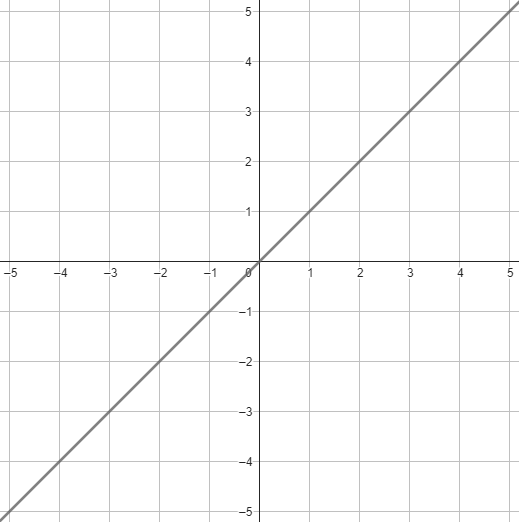Graphs of linear functions are straight lines, that go from top-right to bottom-left, or top-left to bottom-right. Their formula is y = ax + b.
Constant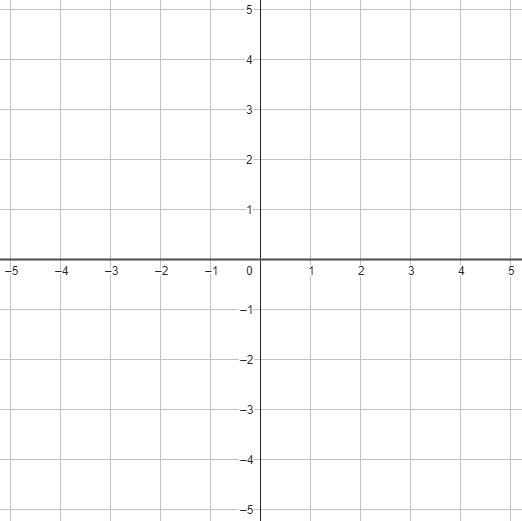Graphs of constant functions are straight lines parallel to the x axis. Their formula is y = a.
Reciprocal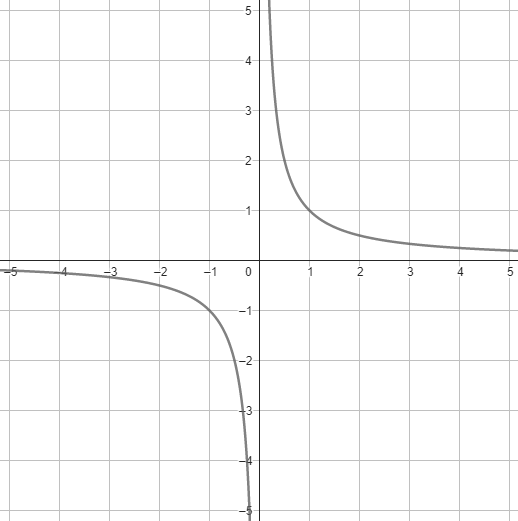Graphs of reciprocal functions form hyperbolas, which are curves with two parts. Their formula is y =
ax
+ b.
Quadratic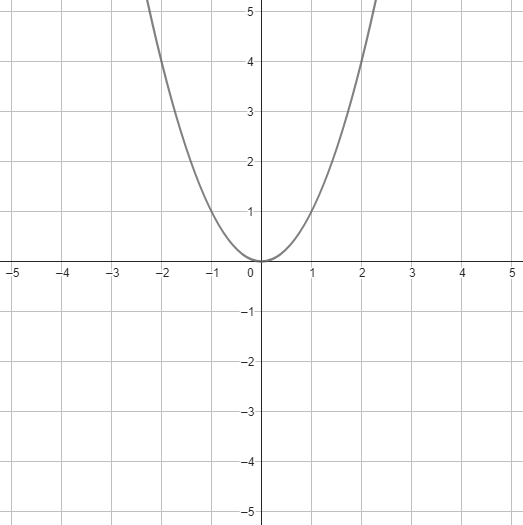Graphs of quadratic are curves called parabolas, which are curves with only one part. Their formula is y = ax3 + bx2 + cx + d.

### Explanation

Type Example image Description
Cubic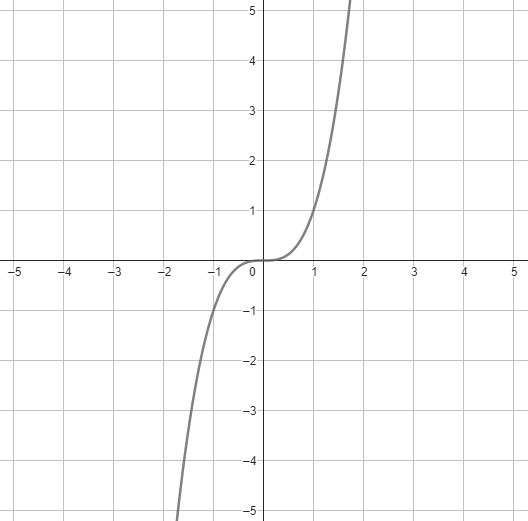Graphs of cubic functions diverge to infinity on one side, and to negative infinity on the other. Their formula is y = ax3 + bx + c.
Goniometric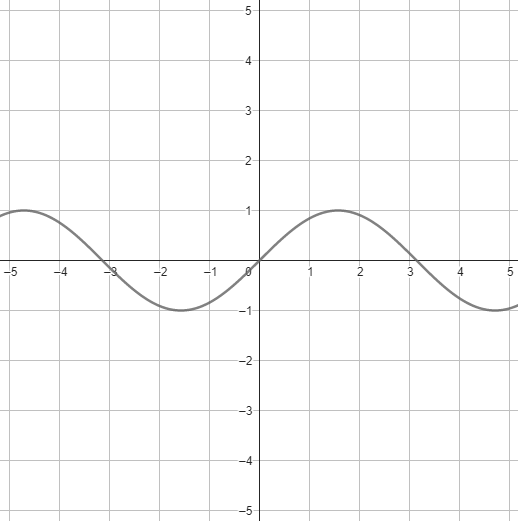Graphs of goniometric functions are odd. They are periodic and non-constant. This module takes their inverses as goniometric functions as well. You will not find any exponencionally growing goniometric functions in this module. Their formula is different depending on which goniometric function they use.
Exponential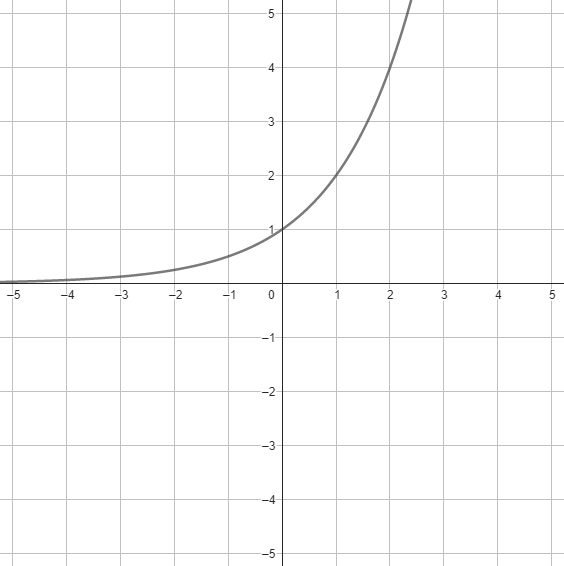Graphs of exponential functions are curves (there is only one curve) that grow exponentially. Their formula is y = ax + b.
Logarithmic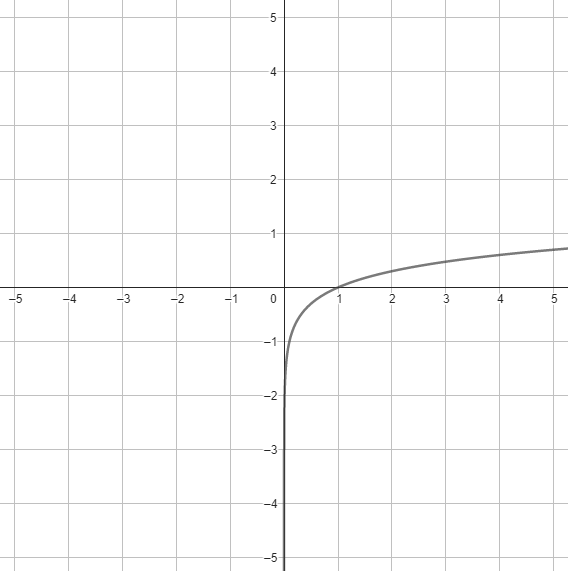Graphs of logarithmic functions are curves very similar to exponential graphs. In fact, logarithmic functions are inverses of exponential functions. Their formula is y = logax + b.

### Properties of graphs

• Graphs can be moved up or down.
• Graphs can be moved left or right.
• Graphs can be flipped along the x and/or y axis.

### Other information

• There are only 10 graphs of each function.
• All the information corresponds to real-life math. Some functions are taught about in elementary school and some in high school.